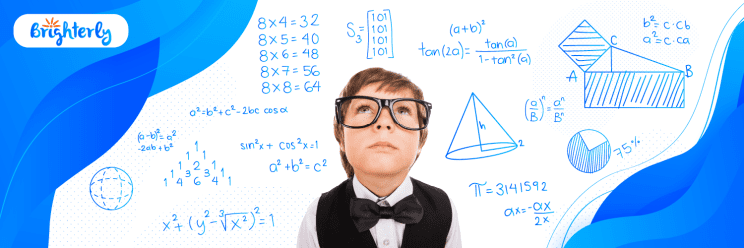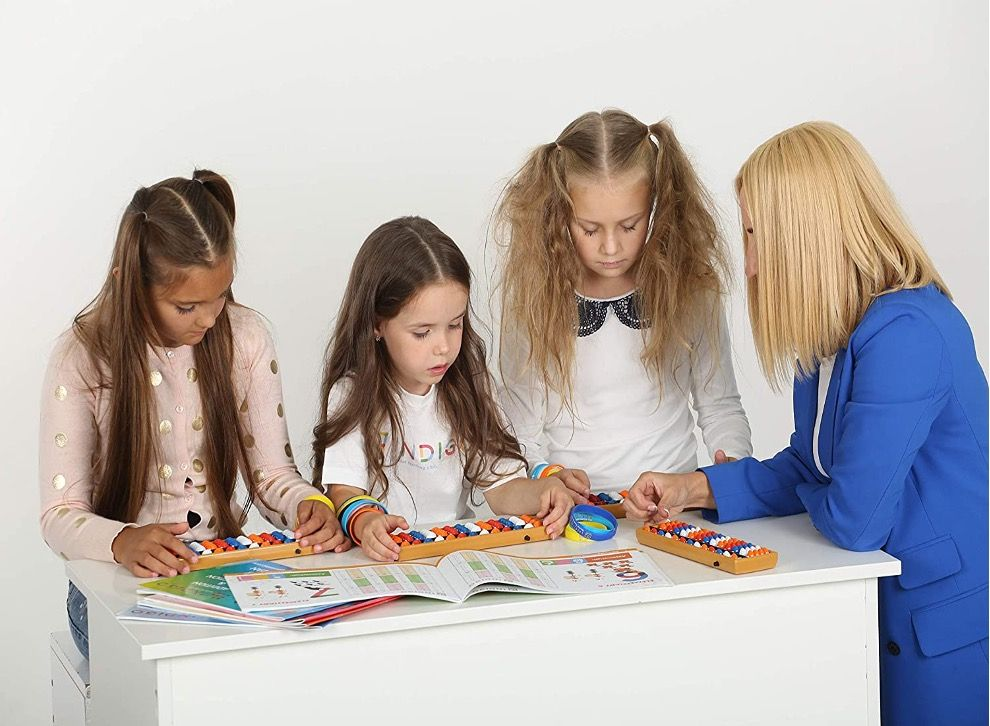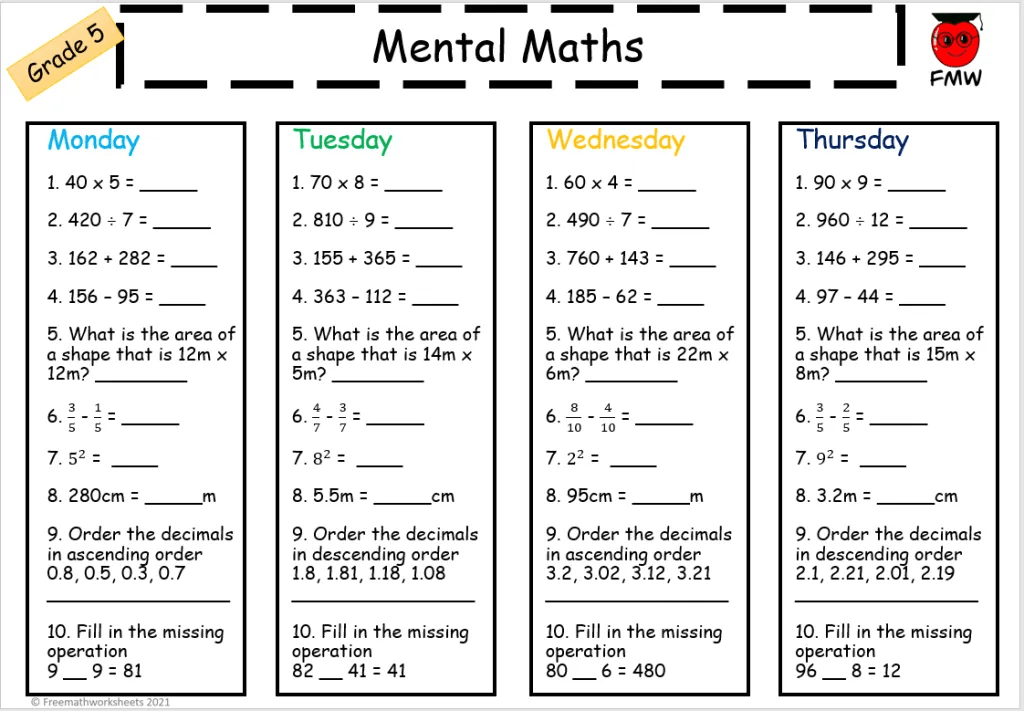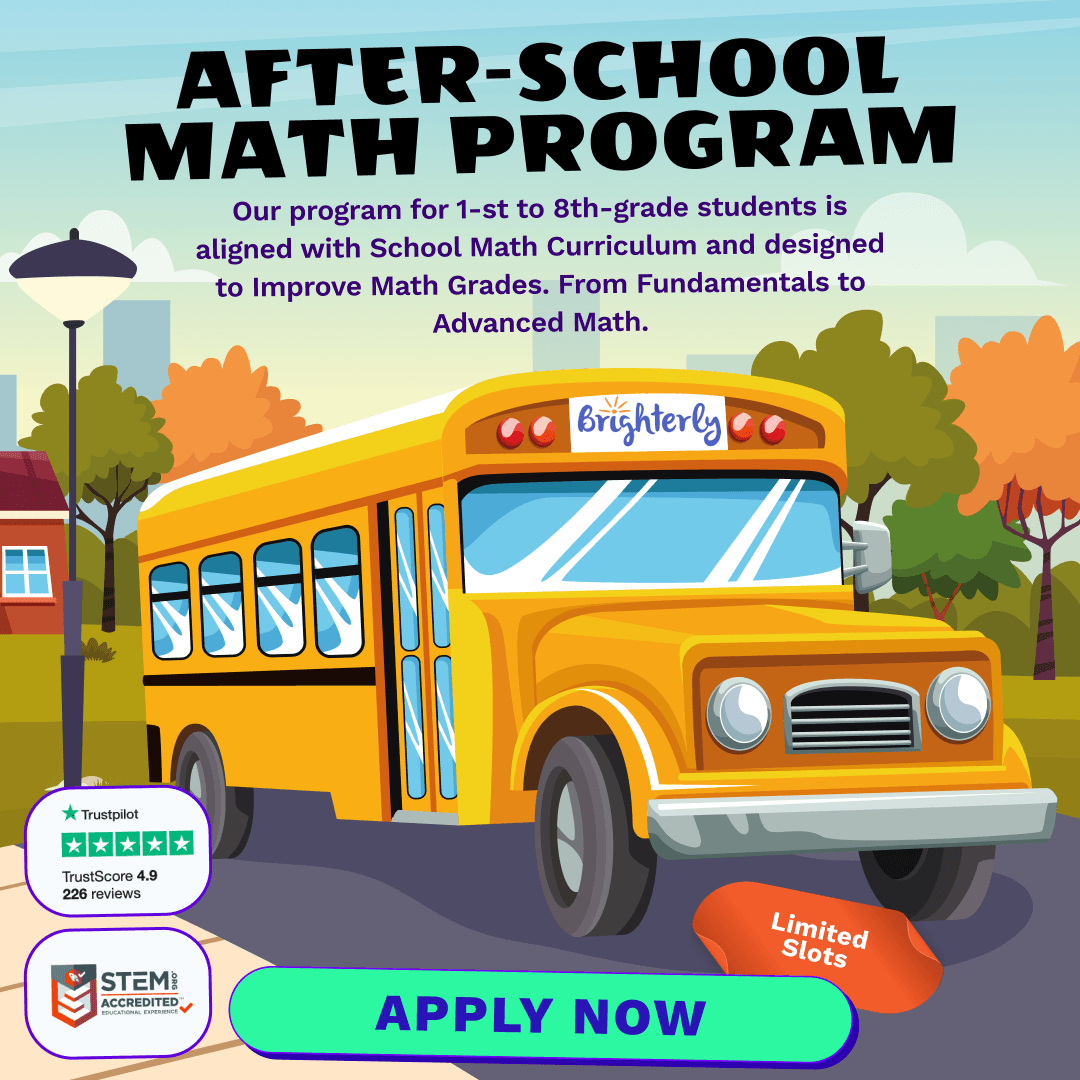# Mental Math Practice Is a Must for Your Kid

ParentingMental math is a great way to keep your mind sharp! Mental math practice improves  overall mathematical skills. In the end, it helps to enhance kids’ counting and verbal arithmetic skills. This article will discuss how to learn mental math and how the practice can improve the ability to solve problems mentally.

## What Is Mental Math?

Mental math is the practice of making mathematical calculations in your head. It is the application of mental math strategies that allows you to perform complex mathematical calculations entirely in your mind, without written or electronic computation.

You can apply mental math in everyday activities such as doing basic math in your head, solving complex equations, and counting money.

Mental math is an important skill that helps kids with everyday calculations, problem-solving, and more complex math (like algebra). While some children are naturally gifted with quick, accurate, and confident mental math abilities, others may need to work harder to develop these skills.

## How to Practice Mental MathMental math is a valuable skill that can help you in everyday life. You can use it to budget your money, calculate tips, and figure out if a sale price is worth it. The best way to learn mental math is to practice regularly. Start with simple calculations like adding numbers up to 10.

Then, practice with larger numbers and start doing more complicated equations. Finally, try to master tricks like estimating and rounding so you can make calculations using mental math techniques more quickly. Here are some suggestions on how to improve mental math skills:

### Practice with a timer

Set aside five minutes each day for mental math practice and use a timer to keep yourself honest. You might get surprised at what you can achieve if you focus on nothing but math for that short amount of time.

### Use flashcards

Flashcards are an old-school favorite for a simple reason — they work! Even better, flashcards help you increase the speed and accuracy of calculations and retention of information.

### Put away the calculator

Avoid using calculators when doing simple tasks like adding up your grocery bill. The more frequently you practice basic arithmetic without assistance, the better you will get at it and the less likely you will rely on calculators in future.

### Practice frequently

If you want to get good at something, you need to practice it often. Mental math is no different.

Do the calculations in your head — don’t write things down or use a calculator. You can keep a piece of scratch paper handy if you need it, but do all your calculations mentally so that you are forced to rely on your brain’s math abilities.

### Focus on the big picture

Visualization strategies include the use of visual images to help you understand and solve problems. They use diagrams, graphs, tools, and spatial reasoning. For example, when we look at 5×6, we might visualize a number line as follows: 5+5+5+5+5+5=30.

### Break down large numbers into smaller numbers

The easiest way to multiply one number by another is by breaking them down into smaller numbers that are easy to multiply separately.

Math for Kids

Is Your Child Struggling With Math?
1:1 Online Math Tutoring### Use estimation and approximation

Mental math isn’t about being perfect; it’s about quickly using strategies to get results that are close enough to the correct ones.

### Use mental shortcuts and tricks

There are many ways to simplify complicated mathematical problems using clever shortcuts and tricks.

### Mental Math Books

Mathematics is an area of study many people shy away from, but some find numbers fun and exciting. There is a wide range of books on mental math from those for kids to college textbooks.

#### Brain Games

It is a great book authored by Brain Games that includes easy-to-understand activities featuring various math practices. The book may be helpful to children in improving their mental math skills. Besides, it is a simple and fun way to practice every day. With this book, kids will be able to solve problems, understand concepts, perform calculations, and just have fun!

Brain Gamesis a book about kids who can’t help but do math – in their heads, with their hands, or with any other method they can think of. The book will help you learn some quick ways to get answers and enjoy studying simultaneously.

Mental Math, Grade 4 helps students master mental calculation strategies for performing addition, subtraction, multiplication, and division in the fourth grade. It is a compelling book published by Frank Schaffer Publications to further students’ understanding of whole numbers, fractions, and decimals.

Furthermore, it helps children succeed in fourth-grade math with a balanced, multi-sensory approach. In the book, math facts appear in a way that makes sense to young students.

#### Mental Math Book 1

It is a complete step-by-step guide written by Bernice Lau Pui Wah. This book teaches kids mental math strategies for addition, subtraction, multiplication, and division. These are the skills that will get kids ahead in life.

There are numerous examples throughout the book and practice problems at the end of each chapter. It is recommended that kids do the practice problems on a separate piece of paper.

### Estimation Games

Estimation is a valuable skill for many reasons. It allows people to quickly size up situations and make decisions like whether or not they have time to make it across the street before the light changes.

• Gain self-confidence
• Make informed decisions

One of the best way to practice mental math is by playing estimation games as highlighted by the following examples:

#### Estimation180 GameIn this game, you have two numbers and ask for an estimation of their addition or subtraction result within 180 seconds. In case of addition, you have to provide the correct result by estimating the sum of those two numbers. In case of subtraction, you have to estimate the difference between two given numbers.

The number of questions asked in this game is 10, and all of them focus on addition and subtraction only. This game is an easy way to practice mental math by adding and subtracting two small numbers.

In this game, the goal is to learn to estimate the total of two or more numbers mentally. You need a calculator, a pen and paper, and a friend willing to cooperate.

How do you play? The first person writes down the number 23 on a piece of paper and passes the paper to the other player who writes another number on the same piece of paper and passes it back.

Keep passing the paper back and forth until one player thinks it’s time to stop. Then calculate the total of all numbers written on the paper so far — call this “N.” Check the correct result with a calculator, and whoever comes closest to estimating N wins!

### Make Use of Math Apps and Websites

Practice mental math online by using math apps and websites. Math is a complicated subject applicable in almost all aspects of our lives. It is one of those subjects that many people find difficult, but others enjoy it thoroughly.

Like for any other subject, there are many tools and apps that you can use to learn mental math strategies better or faster, and while some are very effective, others are not. When learning math, the use of some of the best apps and websites helps you determine which ones you think are the most efficient for your studies.

And more importantly, the role of these online resources is to allow children to understand mental math rather than just being able to complete the mathematical operations mechanically.

They should understand why they are learning and appreciate the importance of math in their daily lives. Math apps will help your children know what they need to work on.

Math for Kids

Is Your Child Struggling With Math?
1:1 Online Math Tutoring#### Benefits of Math Apps and Websites

##### 1. Students Can Work at Their Own Pace

Math apps and websites are a great way to encourage independent learning and increase mental math skills. These tools allow students to work at their own pace, in their own time and develop deeper know-how of the topics they are studying. It is not just about solving equations on your phone, either.

Math apps can help with memorization, visualization, and analysis – all critical skills for developing math fluency. Online resources allow kids to go back over topics they do not understand until they fully comprehend them.

##### 2. Step-by-Step Solutions

Typically, math apps and websites provide step-by-step solutions for each problem which helps students understand why the solution works. Better yet, students have a greater understanding of how to improve mental math skills than simply memorizing formulas without understanding how to use them.

Similarly, students have access to different types of practice problems, which is important because kids are exposed to problem-solving and may even face tasks that are not covered in class.

##### 3. Provide Immediate Feedback

When students are learning mental math, they sometimes get stuck and are unsure what to do next. With a math app or website, they can keep working and trying until they get the right answer. Thus, they remain motivated rather than discouraged.

When students learn to read, they do not always understand what they read. Math is different. You either get the right answer, or you don’t. The “right” answer is concrete and objective. Students can keep working with math apps and websites until they get it right! Beyond that, the method builds confidence like nothing else can!

For example, when a student uses a math app or website, the program will tell them whether their answer is right or wrong. It’s an immediate feedback. If students get the answer right, they can move on. Additionally, most apps and websites also explain what went wrong.

Studying with Brighterly

Brighterly is a new and innovative online learning platform that boasts tutors who provide students with the tools to succeed in their studies.

The Brighterly site offers students lessons on various subjects, including Math. Thus, kids can practice mental math online with experienced teachers. In addition, students can log in from any part of the world and learn from the comfort of their own homes.

### Mnemonic Devices

Mnemonics are memory tools to help students learn and retain new information in mental math. They use vivid imagery and creative spelling to make memorization easier when dealing with mental math games. Mnemonics work by creating associations between small pieces of information, making them easier to be remembered.

Many students have trouble remembering formulas and important details in math. One way to help them memorize these things is through mnemonics. Mnemonics are memory aids that use a string of letters or associations to help you remember something else.

Here are some examples of mnemonic devices in math:

#### a) Acronym

The acronym PEMDAS is useful when remembering the order of operations. PEMDAS stands for Parenthesis (brackets), Exponents, Multiplication and Division, Addition and Subtraction.

These are the steps you follow when evaluating an expression with multiple operations.

Example: Solve this problem: One example of an important order of operations is the equation (3+6)×5+1=?  Using PEMDAS, you would get 46 because parentheses should be solved before multiplication, and addition is the last operation when going from left to right in the equation.

#### b) The Bundles of Sticks Method for MultiplicationIt is one of the most popular methods of multiplication, and you can find it in many books. Let’s take an example 63 x 18 = 1134, which means you have to multiply 63 by 18. Take each digit in 63 and multiply it by 18; so, 3 x 18 = 54 and 60 x 18 = 1080. Then you add the numbers together: 54+1080=1134.

### Mental Math ActivitiesMental math activities are essential to help children connect math to the real world, including the world of numbers. These activities are a fun way to introduce numbers, counting, addition, and subtraction. Kids will love them!

Here are some exciting mental math activities you can try with your child:

Materials

1. Dice (one per person)
2. Number line (optional)
3. A pencil and paper for each person to record their answers

Directions

Roll two dice, one at a time, and add them together. If you want to record your answers on paper, draw a number line between 0 and 12.

Each time you roll two dice and add them together, write the answer on the number line in the box that matches the sum. Such an approach will help your child see the relationship between addition and subtraction. You can also use this activity as an opportunity to introduce or practice place value by using three dice (hundreds, tens, ones).

Another activity is working with mental math practice worksheets, which are easy to find online.## Conclusion

If your kids are struggling with math, mental math practice is for them. Tips from this article can help you double childrens’ math speed and get better at mental math. Learning mental math techniques can take some time, but in the end, it can make one’s life easier.

After-School Math Programs• Our program for 1st to 8th grade students is aligned with School Math Curriculum.

After-School Math Programs
Our program for 1st to 8th grade students is aligned with School Math Curriculum.